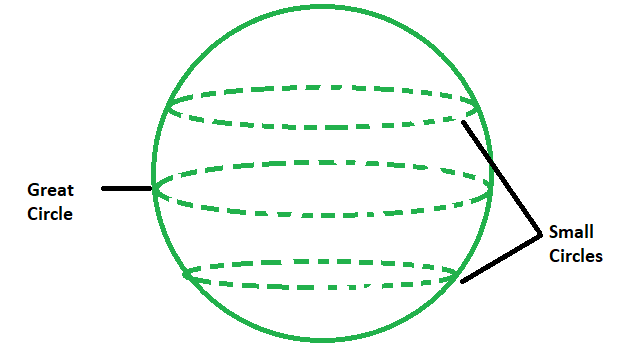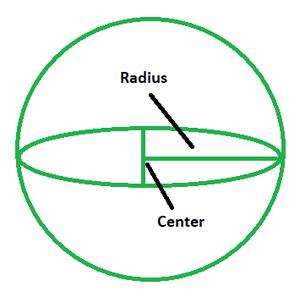Open In App

# Great Circle Distance Formula

The greatest circle that may be drawn on the surface of a sphere is the great circle. A great circle is a region of a sphere that encompasses the sphere’s diameter, and also is the shortest distance between any two places on the sphere’s surface. It is also known as the Romanian Circle.

The figure below depicts a sphere and circles of different radii and sizes that can be drawn on its surface.Application of Great Circle

The application of the great circle formula is utilized in the navigation of aircraft or ships, which is one of the few instances where it is applied. Because the Earth is spherical, the great circle formula aids navigation by determining the shortest distance within the sphere.

As can be seen below, the great circle is the one that shares the centre with the main sphere itself. The diameter of both the sphere and the great circle coincides.### Great Circle Formula

The great circle formula is given as follows:

d = rcos-1[cos a cos b cos(x-y) + sin a sin b]

where,

a and b depict the latitude

while the longitudes are depicted by x and y.

### Sample Problems

Question 1. Given that the radius of a sphere is 4.7 km, latitude being (45°, 32°) and longitude (24°,17°), find the distance of the great circle.

Solution:

The great circle formula is given by: d = rcos-1[cos a cos b cos(x-y) + sin a sin b].

Given: r = 4.7 km or 4700 m, a, b= 45°, 32° and x, y = 24°,17°.

Substituting the values in the above formula, we have:

d = 4700 cos-1(0.52 × 0.83 × 0.75) + (0.85 × 0.32)

= 4700 × 0.99

⇒ d = 4653 m

Question 2. Given that the radius of a sphere is 5 km, latitude being (25°, 34°) and longitude (48°,67°), find the distance of the great circle.

Solution:

The great circle formula is given by: d = rcos-1[cos a cos b cos(x-y) + sin a sin b].

Given: r = 5 km, a, b= 25°, 34° and x, y = 48°,67°.

Substituting the values in the above formula, we have:

d = 5 cos-1(0.913 × 0.83× 0.945) + (0.406 × 0.556)

= 5 × 2.793

⇒ d = 94.928 km or 94928 m

Question 3. Given that the radius of a sphere is 10 km, latitude being (55°, 86°) and longitude (28°,70°), find the distance of the great circle.

Solution:

The great circle formula is given by: d = rcos-1[cos a cos b cos(x-y) + sin a sin b].

Given: r = 10 km, a, b= 55°, 86° and x, y = 28°,70°.

Substituting the values in the above formula, we have:

d = 10 cos-1(0.573 × 0.0707× 0.743) + (0.819 × 0.997)

= 10 × 0.5602

⇒ d = 5.62 km

Question 4. Given that the radius of a sphere is 7 km, latitude being (55°, 86°) and longitude (28°,70°), find the distance of the great circle.

Solution:

The great circle formula is given by: d = rcos-1[cos a cos b cos(x-y) + sin a sin b].

Given: r = 7 km, a, b= 55°, 86° and x, y = 28°,70°.

Substituting the values in the above formula, we have:

d = 7 cos-1(0.573 × 0.0707× 0.743) + (0.819 × 0.997)

= 7 × 0.5602

⇒ d = 3.93 km

Question 5. Given that the radius of a sphere is 4 km, latitude being (25°, 34°) and longitude (48°,67°), find the distance of the great circle.

Solution:

The great circle formula is given by: d = rcos-1[cos a cos b cos(x-y) + sin a sin b].

Given: r = 4 km, a, b= 25°, 34° and x, y = 48°,67°.

Substituting the values in the above formula, we have:

d = 4 cos-1(0.913 × 0.83× 0.945) + (0.406 × 0.556)

= 4 × 2.793

⇒ d = 11.17 km

Question 6. Given that the radius of a sphere is 16 km, latitude being (45°, 32°) and longitude (24°,17°), find the distance of the great circle.

Solution:

The great circle formula is given by: d = rcos-1[cos a cos b cos(x-y) + sin a sin b].

Given: r = 16 km, a, b= 45°, 32° and x, y = 24°,17°.

Substituting the values in the above formula, we have:

d = 16 cos-1(0.52 × 0.83 × 0.75) + (0.85 × 0.32)

= 16 × 0.99

⇒ d = 15.84 km

Question 7. Given that the radius of a sphere is 18 km, latitude being (45°, 32°) and longitude (24°,17°), find the distance of the great circle.

Solution:

The great circle formula is given by: d = rcos-1[cos a cos b cos(x-y) + sin a sin b].

Given: r = 18 km, a, b= 45°, 32° and x, y = 24°,17°.

Substituting the values in the above formula, we have:

d = 18 cos-1(0.52 × 0.83 × 0.75) + (0.85 × 0.32)

= 18 × 0.99

⇒ d = 17.82 km